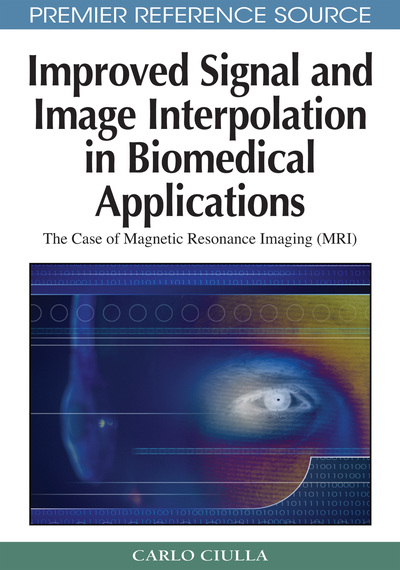# The Results of the Sub-Pixel Efficacy Region Based Bivariate Linear Interpolation Function

Carlo Ciulla (Lane College, USA)
DOI: 10.4018/978-1-60566-202-2.ch008
Available
\$37.50
No Current Special Offers

## Abstract

This is the first of the chapters of the book that present results obtained processing the MRI database with both classic and SRE-based interpolation paradigms. The focus of this chapter is on the bivariate linear interpolation function. An overview on the validation paradigm is given along with an explanation of the simulations that were conducted in order to validate the SRE-based bivariate liner interpolation function. Subsequently, results with real MRI are shown quantitatively through plots of the metric called RMSE Ratio which was employed to assess which one between classic and SRE-based interpolation furnishes lower root-mean-square-error (RMSE). Qualitative demonstration of the results with MRI is also given. The chapter also discusses and evaluates the case of interpolation error improvement when the novel re-sampling location within the pixel is placed on the x axis of the coordinate system (i.e. yr0 = 0) and consistently, both quantitative and qualitative results are presented through plots of the RSME Ratio and figures respectively.
Chapter Preview
Top

Ciulla and Deek (2005) showed quantification of the advantages of the Sub-pixel Efficacy Region (SRE) and also presented the validation paradigm adopted in order to demonstrate the improvement of the bivariate liner interpolation function. For convenience to the reader the metric (RMSE Ratio) employed to assess as to which one of the two model interpolation functions delivers the smallest error is herein recalled along with the procedure employed for its calculation.

The image was misplaced of (x0 y0), then motion correction and interpolation was performed in two different ways: (i) through the classic form of bivariate linear function, and (ii) through the SRE-based bivariate linear function. The latter consists of the classic bivariate interpolation function calculated at the novel re-sampling location. After processing the image with a given misplacement or rotation, the ratio between the two resulting RMSE values was calculated with: (i) the classic bivariate interpolation function (RMSEbefore), and (ii) the SRE-based bivariate interpolation function (RMSEafter) respectively. The ratio was then subtracted from the numerical value of one such to obtain the:

RMSE Ratio = (1 - RMSEbefore / RMSEafter)

A negative value of the RMSE Ratio shows that the SRE-based function outperforms the classic in terms of interpolation error and a positive value shows the opposite.

For the reminder of the book the reader is referred to above definition of RMSE Ratio. This metric is consistently employed in these works to assess the relativity of performance of the pair of interpolation functions: classic and SRE-based.

Ciulla and Deek (2005) reported empirical confirmation of what was foreseen through the two conceptions that set the ground upon which the theory was developed. This was done through the employment of diverse image data among which T1-weighted MRI. The same validation paradigm is herein adopted to further illustrate the results of the application of the Sub-pixel Efficacy Region (SRE) to the bivariate linear interpolation function. This presentation starts with the results obtained on signals of known nature and continues extensively while employing T1-weighted and T2-weighted MRI along with functional MRI data (T2*).

Top

## Simulations

Ciulla and Deek (2005) presented results obtained through simulations performed employing the sin (x, y) function along with the plots of the Intensity-Curvature Functional ΔE after re-sampling and also after motion correction and interpolation through the bivariate linear interpolation function in its classic and the SRE-based forms. The results were corroborated with RMSE plots showing the improvement obtained through the SRE. Also presentation of the behavior of the x and y coordinates of the novel re-sampling locations was given for the cos(x, y) function.

As shown in Figure 1, this section extends the former study and thus presents two more functions: cos (x, y) and ln (x, y). Figures 2 and 3 show the plots of the Intensity-Curvature Functional for re-sampled images (Figure 2) and for re-sampled and motion corrected images (Figure 3).

## Complete Chapter List

Search this Book:
Reset